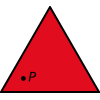You may also likeFitting In

The largest square which fits into a circle is ABCD and EFGH is a square with G and H on the line CD and E and F on the circumference of the circle. Show that AB = 5EF. Similarly the largest equilateral triangle which fits into a circle is LMN and PQR is an equilateral triangle with P and Q on the line LM and R on the circumference of the circle. Show that LM = 3PQTriangle Midpoints

You are only given the three midpoints of the sides of a triangle. How can you construct the original triangle?Three Way Split

Take any point P inside an equilateral triangle. Draw PA, PB and PC from P perpendicular to the sides of the triangle where A, B and C are points on the sides. Prove that PA + PB + PC is a constant.

Altitude Inequalities

Age 14 to 16 ShortChallenge Level

A triangle $T$ has an area of $1$cm$^2$. Let $M$ be the product of the perimeter of $T$ and the sum of the three altitudes of $T$. Which of the following statements is false?

A. There are (or there exist) triangles $T$ for which $M> 1000$
B. $M> 6$ for all triangles $T$
C. There are triangles $T$ for which $M=18$
D. $M> 16$ for all right-angled triangles
E. There are triangles $T$ for which $M< 12$

If you liked this problem, here is an NRICH task which challenges you to use similar mathematical ideas.

This problem is taken from the UKMT Mathematical Challenges.
You can find more short problems, arranged by curriculum topic, in our short problems collection.